25.7 C
New York
Thursday, July 7, 2022
HomeChrome OSHow To Use AND and OR Functions in Google Sheets

# How To Use AND and OR Functions in Google Sheets

We frequently use AND and OR logical functions in Google Sheets. These two logical functions provide you TRUE and FALSE results from multiple arguments based on their criteria. However, AND and OR functions in Google Sheets are easy to apply and get the result out of it when you apply individually.

But, it’s a bit tricky when you combine both the logical functions in one formula. No worries! The post will cover all these things to make you understand easily. Moreover, AND and OR function works both on Excel and Google Sheets.

And, as we all know, Google Sheets is more convenient than Excel nowadays. So, this post is all about the usage of the AND and OR functions in Google Sheets.

## Usage of AND and OR Functions in Google Sheets

There are certain things you’ve to keep in mind before diving into the step-by-step process. You can apply AND and OR functions with as many arguments as you want.

However, to get a TRUE response when you use AND functions with several arguments, all of your arguments need to be true. Else, you’ll get a FALSE response.

But the scenario for the OR function is totally opposite of AND function. That is, to get a TRUE response with the OR function, at least one argument of yours must be true. Otherwise, you’ll get the FALSE response.

Besides, you can apply AND and OR functions individually or with other functions, the IF function, for example. Well, let’s get to the real business.

### 1. Usage of the OR Function In Google Sheets

As I said, the OR function at least requires one argument to be true, unlike the AND function. You can use OR function individually or combine it with another function.

Here’s the OR function with criteria`=OR(Argument A, Argument B)`. However, you can put as many arguments as you want in the function. So, click on any empty cell after opening a Google Sheets, and type the formula accordingly.

Let’s take a look at the below-attached image as an example where the OR function has been applied individually in cell A2.

There are three arguments, and one of the arguments is not true (E4=2, but actually, it’s 19). That is why it results in TRUE.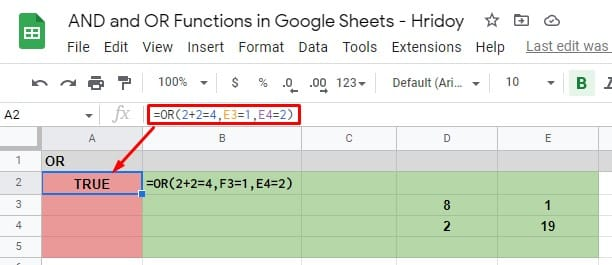And to get the result as FALSE with the OR function, all your arguments need to be incorrect, as I said before. In the example below, A4 and A5 result in FALSE because all the arguments applied there are incorrect.

Since the OR function needs at least one argument true to work, which is not there, that’s why the result comes FALSE.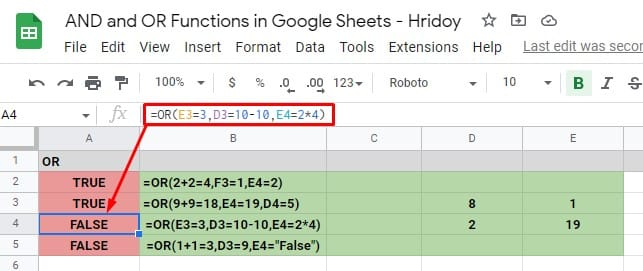### 2. Usage of the AND Function In Google Sheets

Similar to the OR function, you can use AND function individually or combine it with other functions. However, you’ll get TRUE or FALSE results with this AND function as well.

Like before, open a Google Sheets and click on any empty cell. Type this AND function `=AND(Argument A, Argument B)` in the cell and change the arguments as you like.

It’s worth mentioning that there is no limit to using the arguments in the function. As a result, you can use as many as you want, but at least one to work the function properly.

However, in the example attached below, I’ve used three arguments to apply the AND function individually. The first one is D2=4, which is true because the value of D2 in the sheet is actually 4.

The second states that E2=5*9, which is also true. If you multiply 5*9, you’ll get 45 which is the actual value of E2.

The last one urges that the value of E3 (which is 8) is the calculation of 20-12. So, this argument is also true.

Since all the arguments are true here, the AND formula comes with a TRUE response in cell A2. However, if you change any arguments here, you’ll get the FALSE response instead of TRUE then.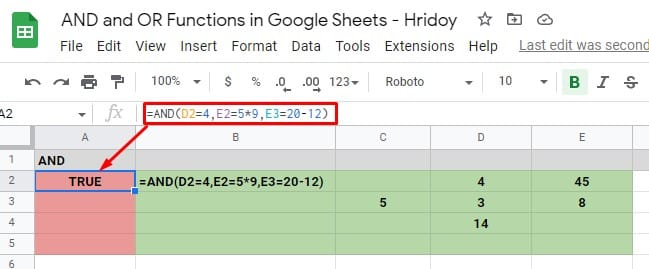In the below example sheets, the AND formula in cell A3 results in FALSE. Because one of its arguments is not true. The last argument says E3=20-13, which means 7, but it’s not correct because the value of E3 is actually 8 in the sheet.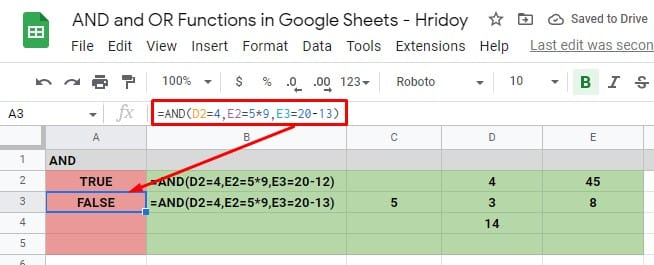## Usage of AND and OR Function with IF

These two logical functions- AND and OR can be used with other functions as their responses TRUE and FALSE. For example, IF. However, by using IF with AND and OR, you can accomplish complex logic.

Having said that, when you use IF with AND and OR, if the arguments are TRUE, it returns one value. If FALSE, it results in the opposite.

### Usage of OR Function with IF

To solve a complex logic text, you can use OR function with IF. Please remember, at least you need to have one correct OR argument to get the TRUE response. Otherwise, you’ll get a FALSE response.

Go to your Google Sheets, click on any cell, and type `=IF (OR(OR Argument 1), Value IF TRUE, Value IF FALSE)`, to use OR function IF.

However, now type the OR arguments as much as you can. Plus, set the values of IF TRUE/IF FALSE as you wish to have in your result.

Now, let’s make things clear with examples. See the below-attached image below first.

In my example sheet, the two OR function with IF (cells A2 and A3) results in the IF TRUE text ‘Yes’. It’s because all three arguments are correct in A2, and one argument is correct in A3 out of four.

On the other hand, all the arguments are incorrect in cell A4. As I said above, you need at least one correct argument in the IF with OR formula to work. So, having an incorrect argument results in IF FALSE text ‘No’ in the sheet.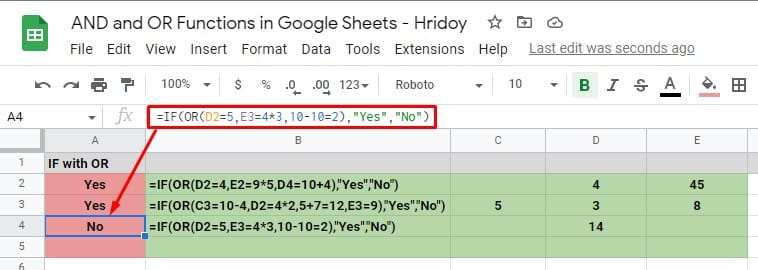### Usage of AND Function with IF

You can use the IF function with AND as well as get a workaround for complex logic. Again stating- all your AND function arguments need to be correct if you want to get a TRUE result.

Well, then click on any cell when you enter Google Sheets. And, type the formula `=IF(AND(AND Argument 1), Value IF TRUE, Value IF FALSE)`. Now, put your AND argument/arguments and IF TRUE/IF FALSE (Yes/No) values.

Now, as you can see in the below image, the IF with AND formula is being applied in cells A2 and A3. The first one, cell A2, results in an IF TRUE ‘Yes’ value because all its arguments are correct.

On the other hand, cell A3 returns with IF FALSE ‘No’ value because of having one incorrect (D3=10+5) argument. D3 contains 14 as a value, where the sum of the arguments will be 15. As a result of this incorrect value, IF FALSE ‘No’ happens.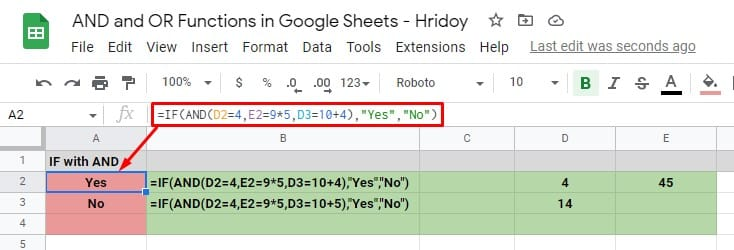## Finally, Insights

To summarize things up, follow the above procedures to apply AND and OR functions in Google Sheets easily. Either you can apply both these logical functions individually or combine them with other functions, like IF, as mentioned above.

Hoping this will be helpful for you. Let me know your feedback in the comment box below. Moreover, sharing this with your near and dear ones will be appreciated much. Well, taking leave now and will be back soon with another Google Workspace hack.Hridoy
Hridoy is a tech enthusiast and loves to do reviews on trending topics like Google docs, sheets, slides, Android ecosystem, etc.